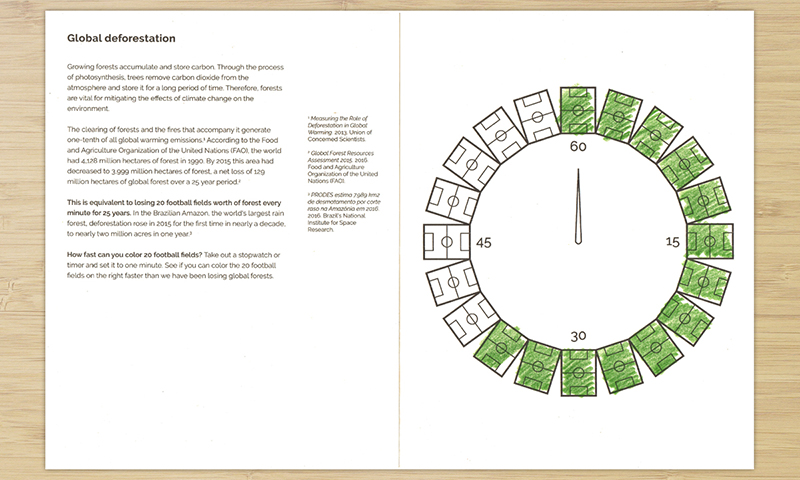# Global deforestation

See if you can color faster than global forests have been destroyed in the past 25 years## Data sources

From the report:

There was a net loss of some 129 million ha of forest between 1990 and 2015, about the size of South Africa, representing an annual net loss rate of 0.13 percent. Yet this should be understood in context: The rate of annual net loss of forest has slowed from 0.18 percent in the 1990s to 0.08 percent over the last five-year period. Between 2010 and 2015 there was an annual loss of 7.6 million ha and an annual gain of 4.3 million ha per year, resulting in a net annual decrease in forest area of 3.3 million ha.

## How the data was used

The following calculations were made to determine the average net number of football fields of global forest lost per minute from 1990 to 2015. First we calculate hectares per minute:

• There are 25 years between 1990 and 2015
• There was a net loss of 129 million hectares of global forest over this time
• That is 5.16 million hectares (`129 / 25`) per year on average
• That is 14,127 hectares (`5.16 / 365.25 x 1,000,000`) per day
• That is 9.81 hectares (`14,127 / 24 / 60`) per minute

Then we determine football fields per hectare:

• A football field is approximately 100 x 50 meters, which is 5,000 square meters
• There are 10,000 square meters in a hectare
• There are 2 football fields (`10,000 / 5,000`) per hectare

Now we calculate loss of football fields per minute:

• `9.8 x 2 = ~20 football fields`

## Code

A python script is available in the code repository to generate or extend this activity, and can be run like this:

``````python deforestation.py
``````

Which will simply create an svg file with the average net number of football fields of global forest lost per minute from 1990 to 2015